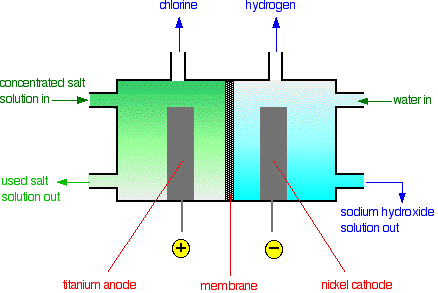# The Manufacture of Chlorine

•• Contributed by Jim Clark
• Former Head of Chemistry and Head of Science at Truro School in Cornwall

This page describes the manufacture of chlorine by the electrolysis of sodium chloride solution using a diaphragm cell and a membrane cell. Both cells rely on the same underlying chemistry, but differ in detail.

## Background chemistry

Chlorine is manufactured by electrolyzing sodium chloride solution. This process generates three useful substances: chlorine, sodium hydroxide and hydrogen.

### The chemistry of the electrolysis process

Sodium chloride solution contains the following:

• sodium ions
• chloride ions
• protons (from the water)
• hydroxide ions (from the water)

The protons and hydroxide ions come from the equilibrium:

$H_2O (l) \rightleftharpoons H^+(aq) + OH^- (aq) \label{1}$

At any time, the concentration of protons or hydroxide ions is very small; the position of equilibrium lies well to the left.

## At the anode

The negative ions, chloride and hydroxide, are attracted to the positively charged anode. It is easier to oxidize hydroxide ions to oxygen than to oxidize chloride ions to chlorine, but there are far more chloride ions arriving at the anode than hydroxide ions.

The major reaction at the anode is therefore:

$2Cl^-_{(aq)}\rightarrow Cl_{2(g)}+2e^- \label{2}$

Two chloride ions each give up an electron to the anode, and the atoms produced combine into chlorine gas. The chlorine is, however, contaminated with small amounts of oxygen because of a reaction involving hydroxide ions, which also give up electrons:

$4OH^-_{(aq)} \rightarrow 2H_2O_{(l)} + O_{2(g)} + 4e^- \label{3}$

The chlorine must be purified by removing this oxygen.

## At the cathode

Sodium ions and protons (from the water) are attracted to the negative cathode. It is much easier for a proton to pick up an electron than for a sodium ion to do so. Therefore, the following reaction occurs:

$2H^+_{(aq)} + 2e^- \rightarrow H_{2(g)} \label{4}$

As protons convert into hydrogen gas, the equilibrium below shifts to the right to replace them:The net effect of this process is a buildup of sodium ions and newly-produced hydroxide ions at the cathode. In other words, sodium hydroxide solution is formed.

## The necessity of keeping all products separate

If chlorine comes into contact with hydrogen, it produces a mixture that explodes violently on exposure to sunlight or heat, producing hydrogen chloride gas. Clearly these gases must remain separated. However, chlorine also reacts with sodium hydroxide solution to produce a mixture of sodium chloride and sodium chlorate(I), also known as sodium hypochlorite; this mixture is commonly sold as bleach. In addition, when the desired products are chlorine and sodium hydroxide rather than bleach, chlorine and sodium hydroxide must also be kept apart. The diaphragm and membrane cells are designed to keep all the products separate.Figure 1: The diaphragm cell

### The diaphragm

The diaphragm is made of a porous mixture of asbestos and polymers. The solution can seep through it from the anode compartment into the cathode compartment. Notice that there is a higher level of liquid on the anode side. This ensures that the liquid always flows from left to right, preventing any of the sodium hydroxide solution from coming into contact with chlorine products.

### Production of chlorine at the anode

Chlorine is produced at the titanium anode according to the following equation:

$2Cl^- (aq) - 2e^- \rightarrow Cl_2(g)$

The product is contaminated with some oxygen because of the reaction below:

$4OH^-(aq) - 4e^- \rightarrow 2H_2O(l) + O_2(g)$

The chlorine is purified by liquefaction under pressure. The oxygen remains a gas when compressed at ordinary temperatures.

### Production of hydrogen at the cathode

Hydrogen is produced at the steel cathode by the following process:

$2H^+ (aq) + 2e^- \rightarrow H_2 (g)$

### Production of the sodium hydroxide

A dilute solution of sodium hydroxide solution is also produced at the cathode (see above for the explanation of what happens at the cathode). It is highly contaminated with unreacted sodium chloride.

The sodium hydroxide solution leaving the cell is concentrated by evaporation. During this process, most of the sodium chloride crystallizes out as solid salt. The salt can be separated, dissolved in water, and passed through the cell again. Even after concentration, sodium hydroxide still contains a small percentage of sodium chloride.Figure 2: The membrane cell

### The membrane

The membrane is made from a polymer that only allows the diffusion of positive ions. That means that the only sodium ions can pass through the membrane; the chloride ions are blocked. The advantage of this is that the sodium hydroxide formed in the right-hand compartment is never contaminated with sodium chloride. The sodium chloride solution must be pure. If it contains any other metal ions, these can also pass through the membrane and so contaminate the sodium hydroxide solution.

### Production of chlorine

Chlorine is produced at the titanium anode according to the following equation:

$2Cl^- (aq) + 2e^- \rightarrow Cl_2(g)$

It is contaminated with some oxygen because of the parallel reaction below:

$4OH^-(aq) + 4e^- \rightarrow 2H_2O(l) + O_2$

The chlorine is purified by liquefaction under pressure. The oxygen remains in the gas phase when compressed at normal temperatures.

### Production of hydrogen

Hydrogen is produced at the nickel cathode as follows:

$2H^+ (aq) +2e^- \rightarrow H_@ (g)$

### Production of sodium hydroxide

An approximately 30% solution of sodium hydroxide solution is also produced at the cathode (see the background chemistry section for an explanation of what happens at the cathode).